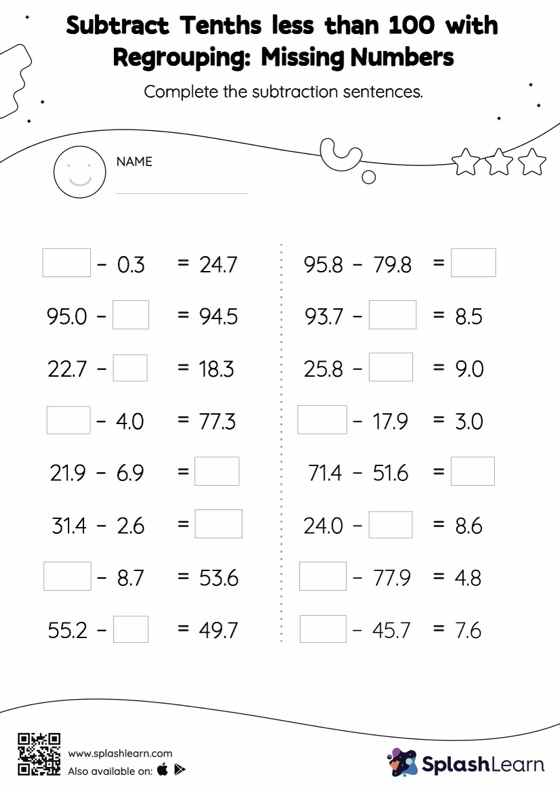# Subtract Tenths less than 100 with Regrouping: Missing Numbers Worksheet

Home > Subtract Tenths less than 100 with Regrouping: Missing NumbersThis worksheet strengthens students' accuracy and strategic flexibility while they subtract tenths less than 100 with regrouping. Students find the missing number while subtracting decimals by using the relationship between addition and subtraction. To reach the answer, they also use the relationship between ones, tenths, hundredths, thousandths, etc., To regroup the numbers in subtract tenths less than 100 with regrouping worksheet.